NumPy数值计算基础（超详细的numpy使用方法）黎明之道1503 0 0

# NumPy数值计算基础

• NumPy是在1995年诞生的Python库Numeric的基础上建立起来的，但真正促使NumPy的发行的是Python的SciPy库。但SciPy中并没有合适的类似于Numeric中的对于基础数据对象处理的功能。于是，SciPy的开发者将SciPy中的一部分和Numeric的设计思想结合，在2005年发行了NumPy。
• NumPy是Python的一种开源的数值计算扩展库。它包含很多功能，如创建n维数组（矩阵）、对数组进行函数运算、数值积分等。 NumPy的诞生弥补了这些缺陷，它提供了两种基本的对象：
• ndarray：是储存单一数据类型的多维数组。
• ufunc：是一种能够对数组进行处理的函数。
• NumPy常用的导入格式：import numpy as np

## 一、NumPy多维数组

### 1 创建数组对象

#### （1）利用array函数创建数组对象

array函数的格式：np.array(object, dtype,ndmin)

array函数的主要参数及说明：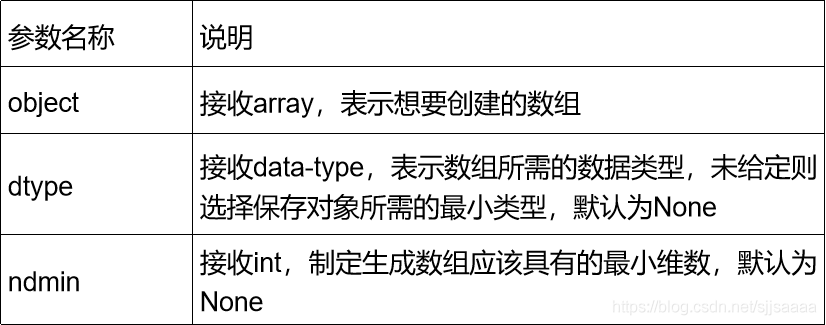``````import numpy as np
data1 = [1,3,5,7] #列表
w1 = np.array(data1)
print('w1:',w1)
data2 = (2,4,6,8) #元组
w2 = np.array(data2)
print('w2:',w2)
data3 = [[1,2,3,4],[5,6,7,8]] #多维数组
w3 = np.array(data3)
print('w3:',w3) ``````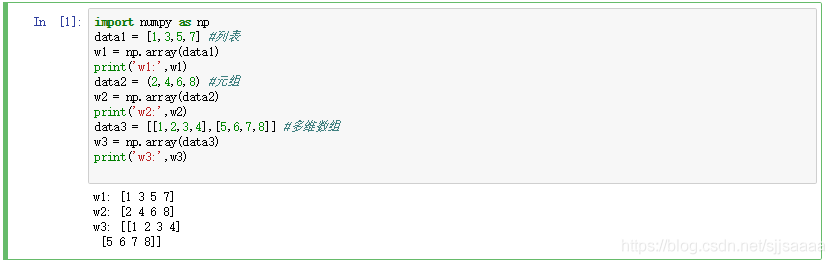#### （2）专门创建数组的函数

• arange函数：创建等差一维数组
• 格式：np.arange([start, ]stop, [step, ]dtype)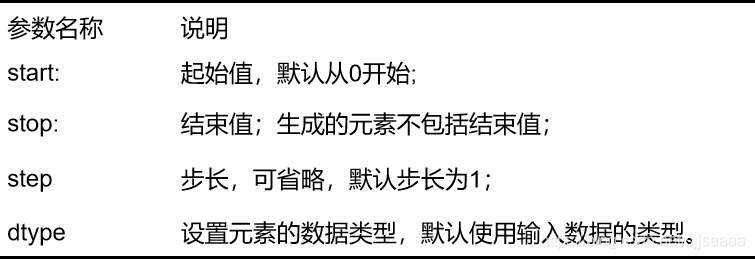``````warray = np.arange(10)
print(warray)
warray = np.arange(0,1,0.2)
print(warray)` ``````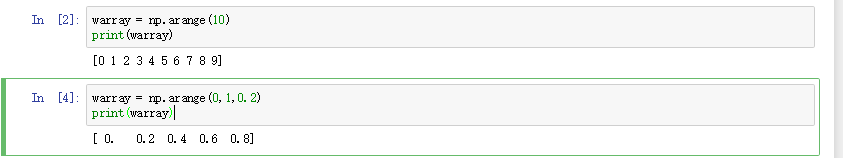• logspace函数：创建等比一维数组
• 格式：np.logspace(start, stop, num, endpoint=True,base=10.0, dtype=None))

logspace的参数中，start, stop代表的是10的幂,默认基数base为10，第三个参数元素个数。

warray = np.logspace(0,1,5)#生成1-10间的5个元素的等比数列
print(warray)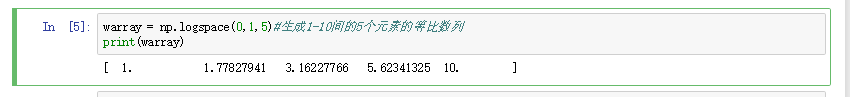• zeros函数：创建指定长度或形状的全0数组
• 格式：np.zeros(shape, dtype=float, order=‘C’)
• ones函数：创建指定长度或形状的全1数组
• 格式：np. ones(shape, dtype=None, order=‘C’)
• diag函数：创建一个对角阵。
• 格式：np.diag(v, k=0)

### 2 ndarray对象属性和数据转换

ndarray对象属性及其说明：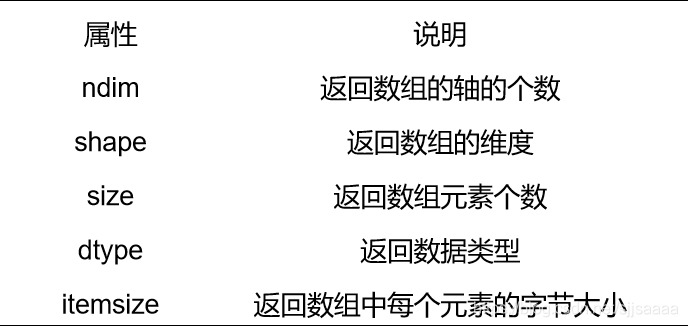``````warray = np.array([[1,2,3],[4,5,6]])
print('秩为：',warray.ndim)
print('形状为：',warray.shape)
print('元素个数为：',warray.size) ``````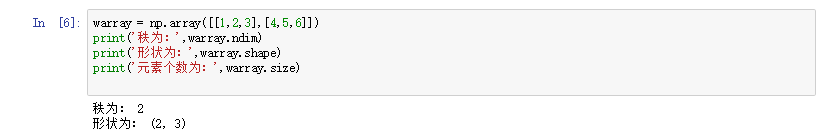``````warray.shape = 3,2
print(warray) ``````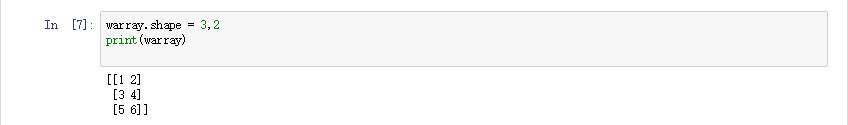``````arr1 = np.arange(6)
print(arr1.dtype)
arr2 = arr1.astype(np.float64)
print(arr2.dtype) ``````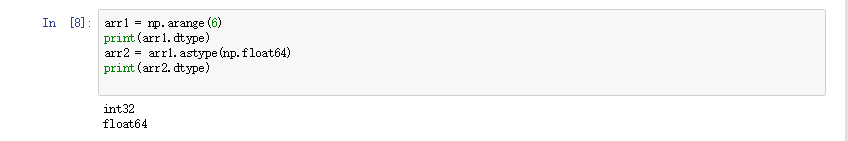### 3 生成随机数

`np.random.randint(low, high = None, size = None)`

``````arr = np.random.randint(100,200,size = (2,4))
print(arr) ``````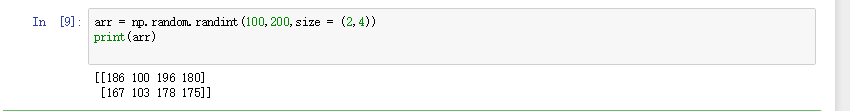random模块的常用随机数生成函数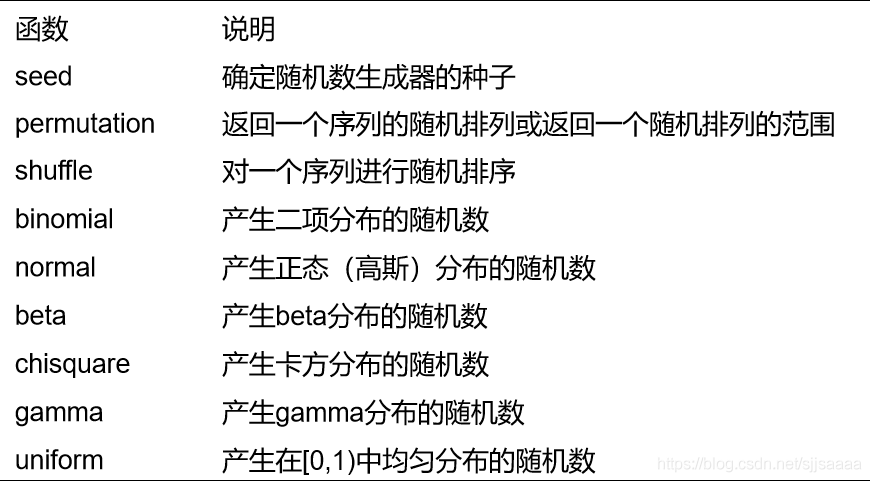### 4 数组变换

#### （1）数组重塑

``````arr1 = np.arange(12).reshape(3,4)
print("arr1:",arr1)
arr2 = arr1.ravel()
print("arr2:",arr2) ``````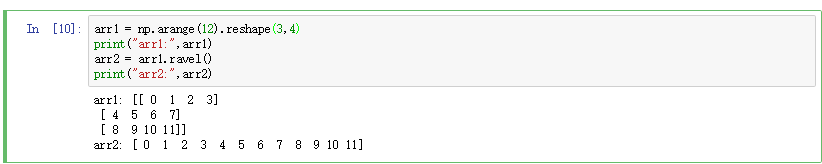#### （2）数组合并

• hstack函数：实现横向合并
• vstack函数：实现纵向组合是利用vstack将数组纵向合并；
• concatenate函数：可以实现数组的横向或纵向合并，参数axis=1时进行横向合并，axis=0时进行纵向合并。
``````arr1 = np.arange(6).reshape(3,2)
arr2 = arr1*2
arr3 = np.hstack((arr1,arr2))
print(arr3) ``````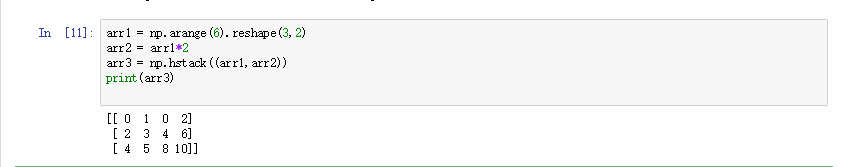#### （3）数组分割

``````arr = np.arange(16).reshape(4,4)
print('横向分割为:\n',np.hsplit(arr,2))
print('纵向组合为:\n',np.vsplit(arr,2)) ``````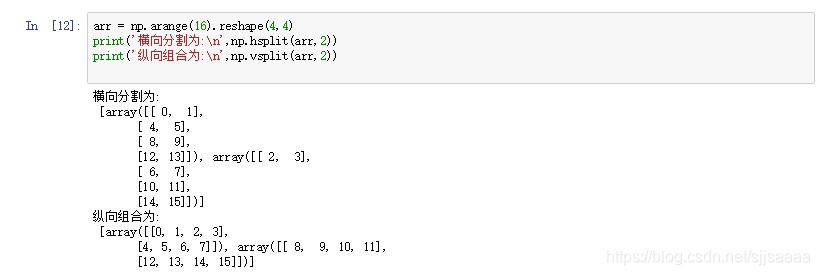#### （4）数组转置和轴对换

``````arr = np.arange(6).reshape(3,2)
print("矩阵：",arr)
print("转置矩阵：",arr.transpose((1,0))) ``````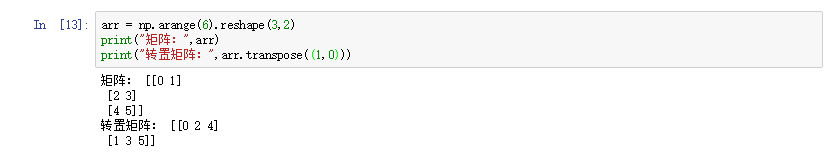## 二、数组的索引和切片

### 1、一维数组的索引类似Python中的列表。

``````arr = np.arange(10)#取1-10
print(arr)#输出arr
print(arr)#输出索引为2的元素
print(arr[-1])#输出最后一个元素
print(arr[1:4])#输出索引为1-3的元素 ``````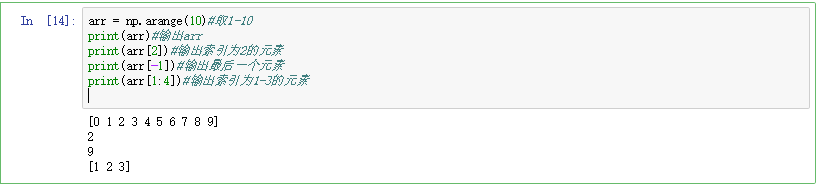``````arr1 = arr[-4:-1].copy()
print(arr)
print(arr1) ``````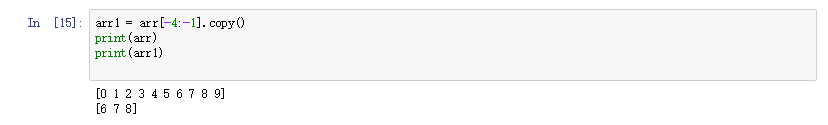### 2、多维数组的索引和切片

• 对于多维数组，它的每一个维度都有一个索引，各个维度的索引之间用逗号分隔。
• 也可以使用整数函数和布尔值索引访问多维数组。
``````arr = np.arange(12).reshape(3,4)
print(arr)
print(arr[0,1:3])  #索引第0行中第1列到第2列的元素
print(arr[:,2])   #索引第2列元素
print(arr[:1,:1])  #第0行第0列元素 ``````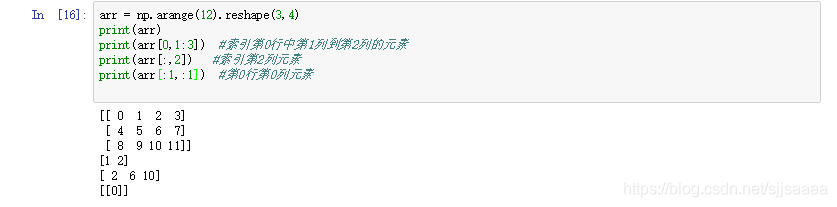``````arr = np.arange(12).reshape(3,4)
#从两个序列的对应位置取出两个整数来组成下标：arr[0,1],arr[1,3]
print(arr)
print('索引结果1：',arr[(0,1),(1,3)])
#索引第1、2行中第0、2、3列的元素
print('索引结果2：',arr[1:2,(0,2,3)])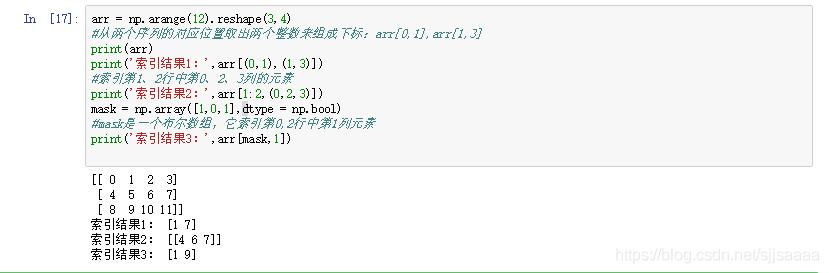## 三、数组的运算

### 1、数组和标量间的运算

``````a = [1,2,3]
b = []
for i in a:
b.append(i*i)

print("b数组:",b)
wy = np.array([1,2,3])
c= wy*2
print("c数组：",c) ``````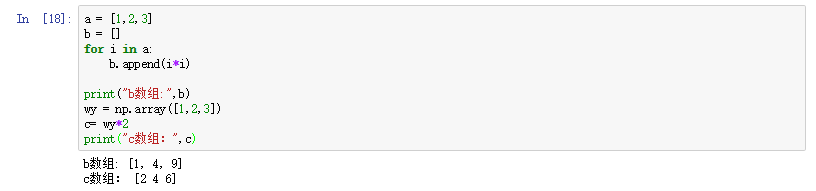### 2、ufunc函数

ufunc函数全称为通用函数，是一种能够对数组中的所有元素进行操作的函数。对一个数组进行重复运算时，使用ufunc函数比使用math库中的函数效率要高很多。

1. 常用的ufunc函数运算

1）四则运算：

2）比较运算：
>、<、==、>=、<=、!=。比较运算返回的结果是一个布尔数组，每个元素为每个数组对应元素的比较结果。

3）逻辑运算：
np.any函数表示逻辑“or”，np.all函数表示逻辑“and”, 运算结果返回布尔值。

``````x = np.array([1,2,3])
y = np.array([4,5,6])
print("数组相加结果：",x+y)
print("数组相减结果：",x-y)
print("数组相乘结果：",x*y)
print("数组幂运算结果：",x**y) ``````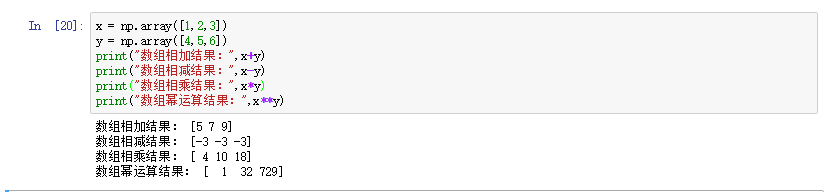``````x = np.array([1,3,6])
y = np.array([2,3,4])
print('比较结果(<)：',x<y)
print('比较结果(>)：',x>y)
print('比较结果(==)：',x==y)
print('比较结果(>=)：',x>=y)
print('比较结果(!=)：',x!=y) ``````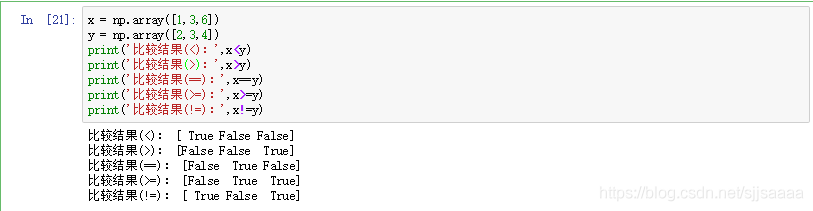1. ufunc函数的广播机制

1）让所有输入数组都向其中shape最长的数组看齐，shape中不足的部分都通过在左边加1补齐。
2）如果两个数组的形状在任何一个维度上都不匹配，那么数组的形状会沿着维度为1的维度进行扩展，以匹配另一个数组的形状。
3）输出数组的shape是输入数组shape的各个轴上的最大值。
4）如果两个数组的形状在任何一个维度上都不匹配，并且没有任何一个维度等于1，则引发异常

``````arr1 = np.array([[0,0,0],[1,1,1],[2,2,2]])
arr2 = np.array([1,2,3])
print("arr1:\n",arr1)
print("arr2:\n",arr2)
print("arr1+arr2:\n",arr1+arr2) ``````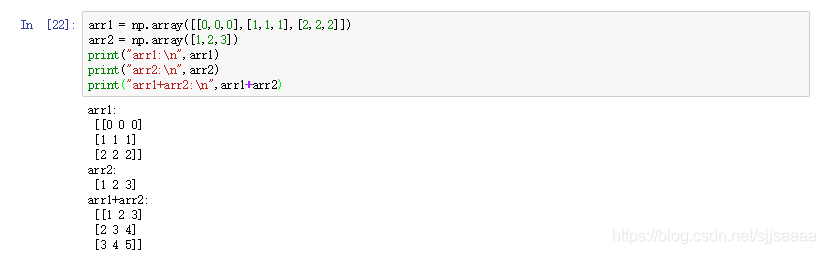### 3、条件逻辑运算

``````arr1 = np.array([1,3,5,7])
arr2 = np.array([2,4,6,8])
cond = np.array([True,False,True,False])
result = [(x if c else y)for x,y,c in zip(arr1,arr2,cond)]
result ``````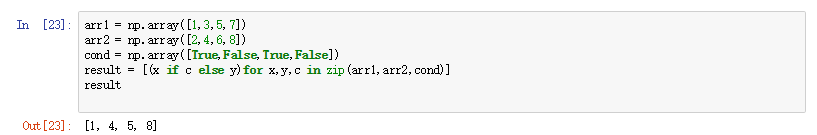where的用法：
np.where(condition, x, y)

``np.where([[True,False], [True,True]],[[1,2], [3,4]],[[9,8], [7,6]]) ``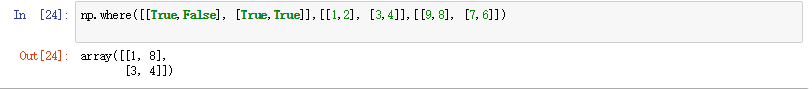``````w = np.array([2,5,6,3,10])
np.where(w>4) ``````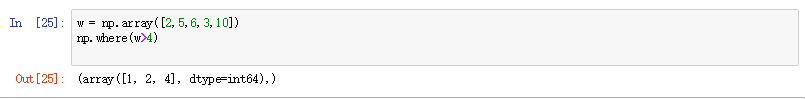where中若只有条件 (condition)，没有x和y，则输出满足条件元素的坐标。这里的坐标以tuple的形式给出，通常原数组有多少维，输出的tuple中就包含几个数组，分别对应符合条件元素的各维坐标。

## 四、数组读写

### 1、读写二进制文件

• NumPy提供了多种文件操作函数存取数组内容。
• 文件存取的格式分为两类：二进制和文本。而二进制格式的文件又分为NumPy专用的格式化二进制类型和无格式类型。
• NumPy中读写二进制文件的方法有：
1. np.save(“文件名[.npy]", arr) 是以二进制格式保存数据。

``````a = np.arange(1,13).reshape(3,4)
print(a)
np.save('arr.npy', a)      # np.save("arr", a)
print(c) ``````

``````a = np.array([[1,2,3],[4,5,6]])
b = np.arange(0, 1.0, 0.1)
c = np.sin(b)   #长度为10
print(c)
np.savez('result.npz', a, b, sin_array = c)
r['arr_0']   #数组a ``````

### 2、读写文本文件

NumPy中读写文本文件的主要方法有：

2. np.savetxt("…/tmp/arr.txt", arr, fmt = “%d”, delimiter = “,”)是将数组写到某种分隔符隔开的文本文件中；
3. np.genfromtxt("…/tmp/arr.txt", delimiter = “,”)是结构化数组和缺失数据。
``np.loadtxt(fname, dtype=, comments=’#’, delimiter=None, converters=None, skiprows=0, usecols=None, unpack=False, ndmin=0, encoding=‘bytes’) ``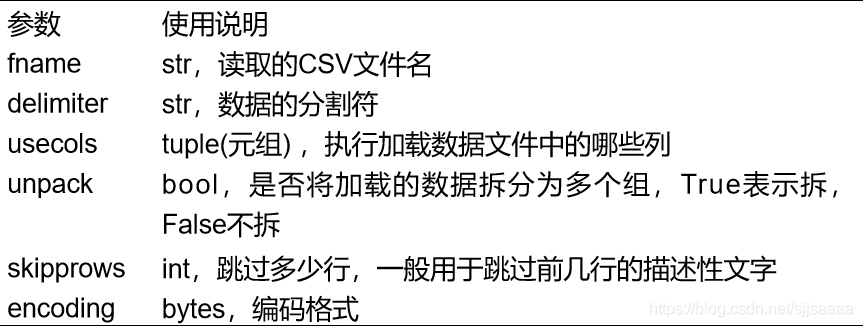## 五、NumPy中的数据统计与分析

### 1、排序

Sort函数对数据直接进行排序，调用改变原始数组，无返回值。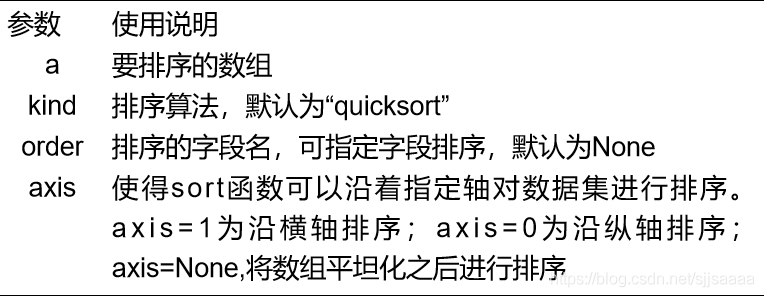• np.argsort函数和np.lexsort函数根据一个或多个键值对数据集进行排序。
• np.argsort(): 返回的是数组值从小到大的索引值;
• np.lexsort(): 返回值是按照最后一个传入数据排序的结果。
``````arr = np.array([7,9,5,2,9,4,3,1,4,3])
print('原数组：',arr)
arr.sort()
print('排序后：',arr) ``````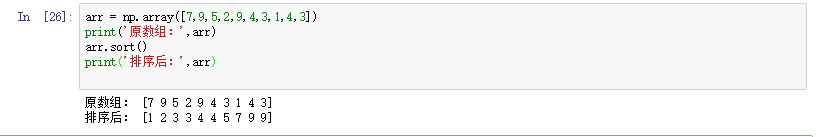``````arr = np.array([[4,2,9,5],[6,4,8,3],[1,6,2,4]])
print('原数组：\n',arr)
arr.sort(axis = 1)   #沿横向排序
print('横向排序后：\n',arr) ``````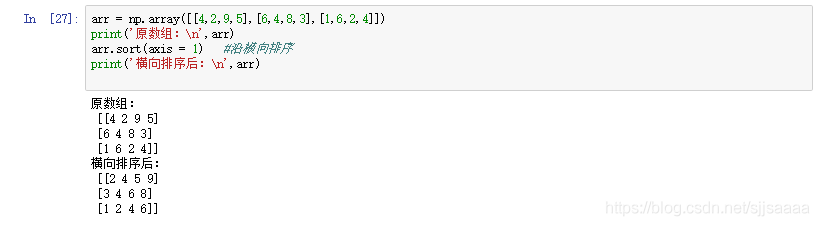``````arr = np.array([7,9,5,2,9,4,3,1,4,3])
print('原数组：',arr)
print('排序后：',arr.argsort())
#返回值为数组排序后的下标排列 ``````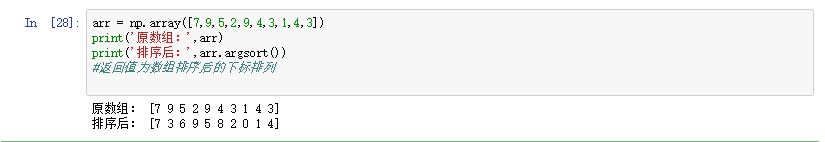``````a = np.array([7,2,1,4])
b = np.array([5,2,6,7])
c = np.array([5,2,4,6])
d = np.lexsort((a,b,c))
print('排序后：',list(zip(a[d],b[d],c[d]))) ``````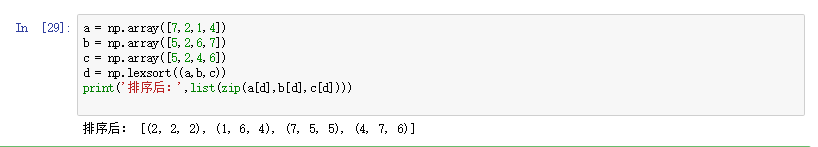### 2、重复数据与去重

``````names = np.array(['红色','蓝色','黄色','白色','红色'])
print('原数组：',names)
print('去重后的数组：',np.unique(names)) ``````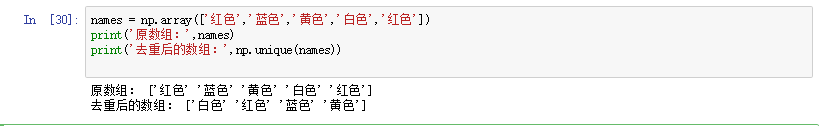• tile函数的格式：np.tile(A, reps)
其中，参数A表示要重复的数组，reps表示重复次数。

• repeat函数的格式：np.repeat(A, reps, axis = None)
“a”: 是需要重复的数组元素，
“repeats”: 是重复次数，
“axis”: 指定沿着哪个轴进行重复，axis = 0表示按行进行元素重复；axis = 1表示按列进行元素重复。

``````arr = np.arange(5)
print('原数组：',arr)
wy = np.tile(arr,3)
print('重复数据处理：\n',wy) ``````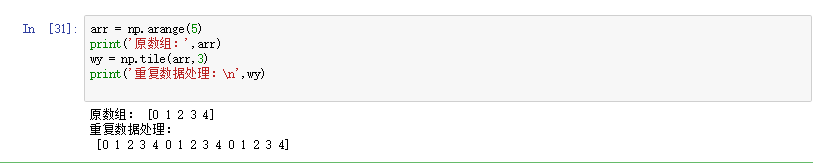``````arr = np.arange(5)
print('原数组：',arr)
wy = np.tile(arr,3)
print('重复数据处理：\n',wy)
arr2 = np.array([[1,2,3],[4,5,6]])
print('重复数据处理：\n',arr2.repeat(2,axis=0)) ``````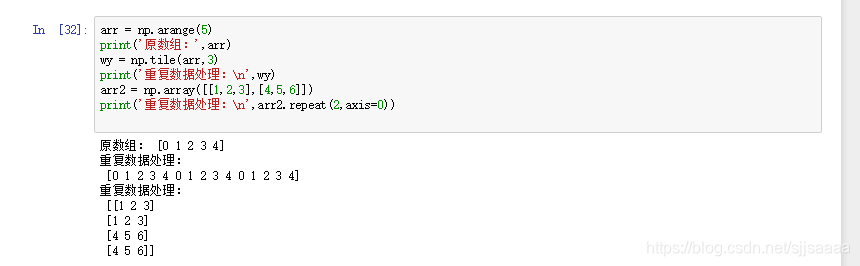### 3、常用统计函数

NumPy中提供了很多用于统计分析的函数，常见的有sum、mean、std、var、min和max等。

axis=0时表示沿着纵轴进行计算，axis=1时沿横轴进行计算。

``````arr = np.arange(20).reshape(4,5)
print('创建的数组：\n',arr)
print('数组的和：',np.sum(arr))
print('数组纵轴的和：',np.sum(arr,axis = 0))
print('数组横轴的和：',np.sum(arr,axis = 1))
print('数组的均值：',np.mean(arr))
print('数组横轴的均值：',np.mean(arr,axis = 1))
print('数组的标准差：',np.std(arr))
print('数组横轴的标准差：',np.std(arr,axis = 1)) ``````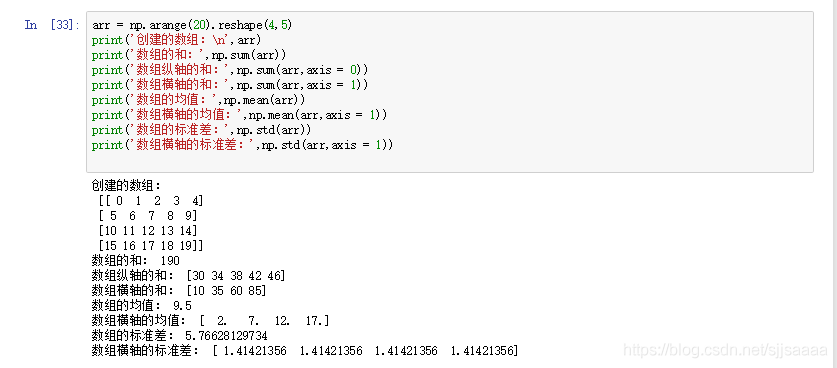### 相关推荐

NumPy数值计算基础（超详细的numpy使用方法）
NumPy数值计算基础 NumPy是在1995年诞生的Python库Numeric的基础上建立起来的，但真正促使NumPy的发行的是Python的SciPy库。但SciPy中并没有合适的类似于Numeric中的对
Pandas统计分析基础（基础篇，新手必看）
Pandas统计分析基础Pandas（Python Data Analysis Library）是基于NumPy的数据分析模块，它提供了大量标准数据模型和高效操作大型数据集所需的工具，可以说Pandas是使得Pyth

Python矩阵和Numpy数组的那些事儿

Ubuntu16.04下安装opencv3.4.2
1.安装官方给的opencv依赖包 * GCC 4.4.x or later * CMake 2.6 or higher * Git * GTK+2.x or higher, including headers (libgtk2.0-dev) # 控制opencv GUI * pkg-config * Python 2.6 or l
Ubuntu16.04安装视觉SLAM环境（OpenCV）
### **一、****安装依赖库** 1 sudo apt-get install build-essential 2 sudo apt-get install cmake git libgtk2.0-dev pkg-config libavcodec-dev libavformat-dev libswscale-dev
Ubuntu16.4下QT配置opencv3.1+FFmpeg

Ubuntu18.04安装OpenCV4.1.0
Ubuntu18.04安装OpenCV4.1.0 1.首先要安装依赖 sudo apt-get install build-essential \ cmake git libgtk2.0-dev pkg-config libavcodec-dev libavformat-dev libswscale-dev \ python-de
ubuntu16.04搭建opencv2.4.13开发环境
1.先去官网下载对应版本的opencv压缩文件,打开终端解压文件. unzip opencv-2.4.13.zip   mv opencv-2.4.13 opencv  2.安装一些必须的依赖库 sudo apt install cmake  sudo apt install build-essential cmake libgtk2.0-dev
Numpy入门（一）：Numpy的安装和创建

Python NumPy学习总结